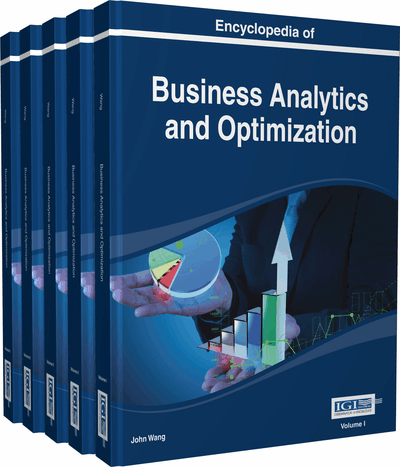# Linear Programming

Xiaofeng Zhao (University of Mary Washington, USA)
DOI: 10.4018/978-1-4666-5202-6.ch127

Top

## Introduction

Linear programming (LP or linear optimization) deals with the problem of the optimization (minimization or maximization), in which a linear objective function is optimized subject to a set of linear constraints. Its name means that planning (programming) is being done with a mathematical model. It is one of widely used techniques in operations research and management science. Some typical applications are: 1. a manufacture wants to develop a production schedule and an inventory policy that will satisfy sales demand in future periods. Ideally, the schedule and policy will enable the company to satisfy demand and at the same time minimize the total production and inventory costs. 2. A financial analyst must select an investment portfolio from a variety of stock and bond investment alternatives. The analyst would like to establish the portfolio that maximizes the return on investment. 3. A marketing manager wants to determine how best to allocate a fixed advertising budget among alternative advertising media such as radio, television, newspaper, and magazine. The manager would like to determine the media mix that maximizes advertising effectiveness. 4. A company has warehouse in a number of locations throughout a country. For a set of customer demands, the company would like to determine how much each warehouse should ship to each customer so that total transport costs are minimized. These examples are only a few of situations in which linear programming has been used successfully, but illustrate the diversity of linear programming applications (Anderson et al., 2011). Other applications include supply chain management in the motor industry, productions scheduling in the brewing industry, aircraft crew and production scheduling, financial planning and capital budgeting, asset and liability management, energy management in the utilities sector, network design in the telecommunications sector and transportation (Dowman & Wilson, 2002).

We are given an m-vector, b = (, . . .,), an n-vector, c = (, . . .,), and an m × n matrix A, whererepresents the vector of decision variables (to be determined), c and b are vectors of coefficients, A is a matrix of coefficients, andis the matrix transpose. The expression to be maximized or minimized is called the objective function (in this case). The inequality A≤ b is the constraint which specifies a convex polytope over which the objective function is to be optimized. The column vector b which is referred to as the right-hand-side vector represents the maximal requirements to be satisfied. A set of variablessatisfying all the constraints is called feasible point or a feasible vector. The set of all such points constitutes the feasible region. Using the foregoing terminology, the linear programming problem can be stated as follows: among all feasible regions, find one that minimizes (or maximize) the objective function. It is fairly common for large linear programming models to include a mixture of functional constraints, some with “ ≤ ”signs, some with “ ≥ ”signs, and some with “ =” signs.

## Key Terms in this Chapter

Slack: The amount of scarce resource of capacity that will be unused by a give feasible solution to a linear programming problem.

Range of Optimality: The range of values over which an objective function coefficient may vary without causing any change in the values of the decision variables in the optimal solution.

Constraint: A restriction that must be satisfied by a solution. It is an equation or inequality that rules out certain combinations of decision variables as feasible solution.

Range of Feasibility: The range of values over which the dual value is applicable.

Unbounded: If the value of the solution may be made infinitely large in a maximization linear programming problem or infinitely small in a minimization problem without violating any of the constraints, the problem is said to be unbounded.

Objective Function: A mathematical expression that describes the problem’s objective.

Decision Variables: A variable that is under the control of the decision maker for a linear programming model.

Dual Value: The change in the value of the objective function per unit increase in the right-hand side of a constraint.

Surplus: A variable subtracted from the left-hand side of a greater-than-or-equal to constraint to convert the constraints into equality. It is the amount by which the constraint is exceeded by a given solution.

Infeasibility: The situation in which no solution to the linear programming problem satisfies all the constraints.

## Complete Chapter List

Search this Book:
Reset